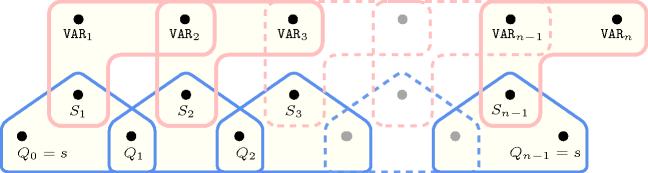## 5.380. strictly_increasing

Origin

KOALOG

Constraint

$\mathrm{𝚜𝚝𝚛𝚒𝚌𝚝𝚕𝚢}_\mathrm{𝚒𝚗𝚌𝚛𝚎𝚊𝚜𝚒𝚗𝚐}\left(\mathrm{𝚅𝙰𝚁𝙸𝙰𝙱𝙻𝙴𝚂}\right)$

Argument
 $\mathrm{𝚅𝙰𝚁𝙸𝙰𝙱𝙻𝙴𝚂}$ $\mathrm{𝚌𝚘𝚕𝚕𝚎𝚌𝚝𝚒𝚘𝚗}\left(\mathrm{𝚟𝚊𝚛}-\mathrm{𝚍𝚟𝚊𝚛}\right)$
Restriction
$\mathrm{𝚛𝚎𝚚𝚞𝚒𝚛𝚎𝚍}$$\left(\mathrm{𝚅𝙰𝚁𝙸𝙰𝙱𝙻𝙴𝚂},\mathrm{𝚟𝚊𝚛}\right)$
Purpose

The variables of the collection $\mathrm{𝚅𝙰𝚁𝙸𝙰𝙱𝙻𝙴𝚂}$ are strictly increasing.

Example
$\left(〈1,3,6,8〉\right)$

The $\mathrm{𝚜𝚝𝚛𝚒𝚌𝚝𝚕𝚢}_\mathrm{𝚒𝚗𝚌𝚛𝚎𝚊𝚜𝚒𝚗𝚐}$ constraint holds since $1<3<6<8$.

Typical
$|\mathrm{𝚅𝙰𝚁𝙸𝙰𝙱𝙻𝙴𝚂}|>2$
Symmetry

One and the same constant can be added to the $\mathrm{𝚟𝚊𝚛}$ attribute of all items of $\mathrm{𝚅𝙰𝚁𝙸𝙰𝙱𝙻𝙴𝚂}$.

Arg. properties

Contractible wrt. $\mathrm{𝚅𝙰𝚁𝙸𝙰𝙱𝙻𝙴𝚂}$.

Counting
 Length ($n$) 2 3 4 5 6 7 8 9 10 Solutions 3 4 5 6 7 8 9 10 11

Number of solutions for $\mathrm{𝚜𝚝𝚛𝚒𝚌𝚝𝚕𝚢}_\mathrm{𝚒𝚗𝚌𝚛𝚎𝚊𝚜𝚒𝚗𝚐}$: domains $0..n$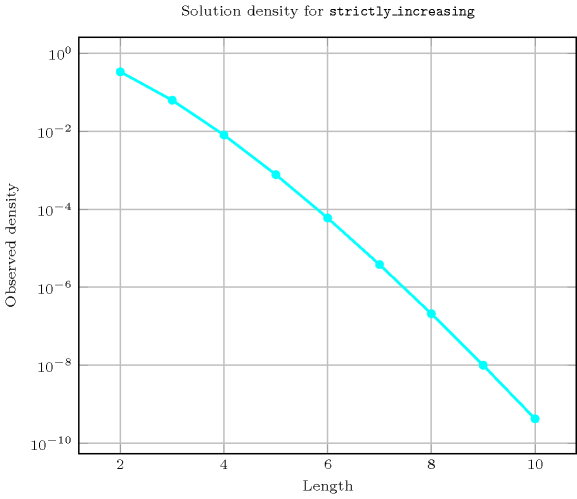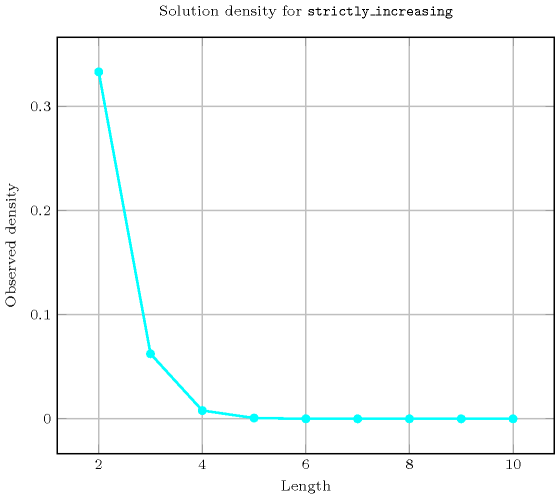Systems
Used in
Keywords
Arc input(s)

$\mathrm{𝚅𝙰𝚁𝙸𝙰𝙱𝙻𝙴𝚂}$

Arc generator
$\mathrm{𝑃𝐴𝑇𝐻}$$↦\mathrm{𝚌𝚘𝚕𝚕𝚎𝚌𝚝𝚒𝚘𝚗}\left(\mathrm{𝚟𝚊𝚛𝚒𝚊𝚋𝚕𝚎𝚜}\mathtt{1},\mathrm{𝚟𝚊𝚛𝚒𝚊𝚋𝚕𝚎𝚜}\mathtt{2}\right)$

Arc arity
Arc constraint(s)
$\mathrm{𝚟𝚊𝚛𝚒𝚊𝚋𝚕𝚎𝚜}\mathtt{1}.\mathrm{𝚟𝚊𝚛}<\mathrm{𝚟𝚊𝚛𝚒𝚊𝚋𝚕𝚎𝚜}\mathtt{2}.\mathrm{𝚟𝚊𝚛}$
Graph property(ies)
$\mathrm{𝐍𝐀𝐑𝐂}$$=|\mathrm{𝚅𝙰𝚁𝙸𝙰𝙱𝙻𝙴𝚂}|-1$

Graph model

Parts (A) and (B) of Figure 5.380.1 respectively show the initial and final graph associated with the Example slot. Since we use the $\mathrm{𝐍𝐀𝐑𝐂}$ graph property, the arcs of the final graph are stressed in bold.

##### Figure 5.380.1. Initial and final graph of the $\mathrm{𝚜𝚝𝚛𝚒𝚌𝚝𝚕𝚢}_\mathrm{𝚒𝚗𝚌𝚛𝚎𝚊𝚜𝚒𝚗𝚐}$ constraint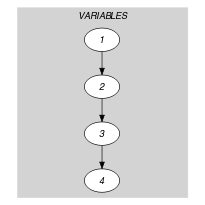(a) (b)
Automaton

Figure 5.380.2 depicts the automaton associated with the $\mathrm{𝚜𝚝𝚛𝚒𝚌𝚝𝚕𝚢}_\mathrm{𝚒𝚗𝚌𝚛𝚎𝚊𝚜𝚒𝚗𝚐}$ constraint. To each pair of consecutive variables $\left({\mathrm{𝚅𝙰𝚁}}_{i},{\mathrm{𝚅𝙰𝚁}}_{i+1}\right)$ of the collection $\mathrm{𝚅𝙰𝚁𝙸𝙰𝙱𝙻𝙴𝚂}$ corresponds a 0-1 signature variable ${S}_{i}$. The following signature constraint links ${\mathrm{𝚅𝙰𝚁}}_{i}$, ${\mathrm{𝚅𝙰𝚁}}_{i+1}$ and ${S}_{i}$: ${\mathrm{𝚅𝙰𝚁}}_{i}\ge {\mathrm{𝚅𝙰𝚁}}_{i+1}⇔{S}_{i}$.

##### Figure 5.380.2. Automaton of the $\mathrm{𝚜𝚝𝚛𝚒𝚌𝚝𝚕𝚢}_\mathrm{𝚒𝚗𝚌𝚛𝚎𝚊𝚜𝚒𝚗𝚐}$ constraint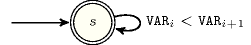##### Figure 5.380.3. Hypergraph of the reformulation corresponding to the automaton of the $\mathrm{𝚜𝚝𝚛𝚒𝚌𝚝𝚕𝚢}_\mathrm{𝚒𝚗𝚌𝚛𝚎𝚊𝚜𝚒𝚗𝚐}$ constraint